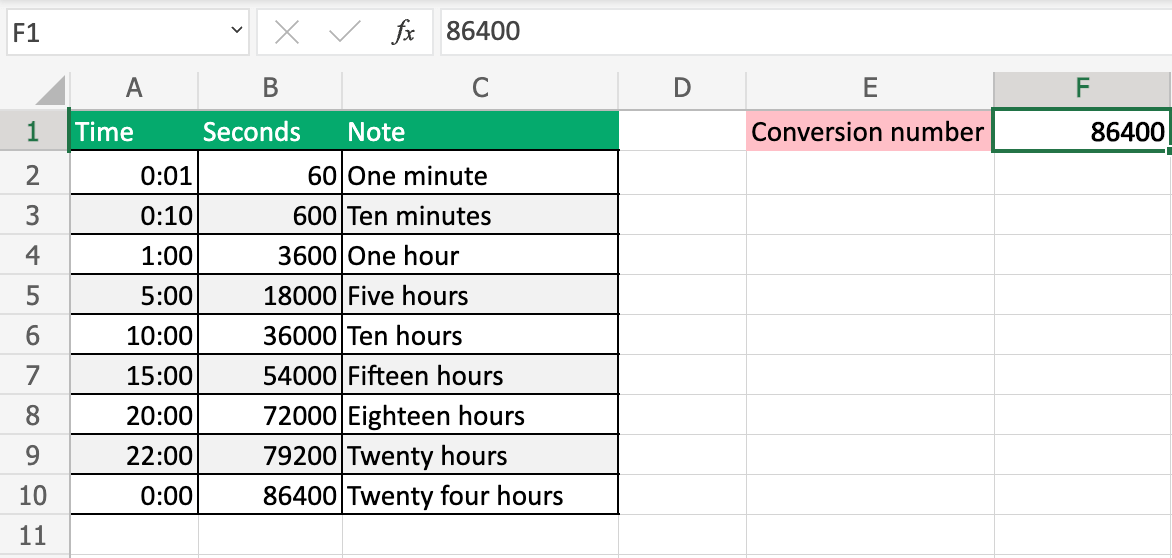# How to Convert Time to Seconds using Excel

Learn how to convert time to Seconds using Excel.

Time can be converted into seconds using Excel.

The number `86400` can be used to convert time to seconds.## Explanation

Each day has 24 hours.

Each hour can be understood as a percentage of the total hours of the day.

• 01:00 = 1/24
• 02:00 = 2/24
• 03:00 = 3/24
• and so on.

The percentage can be used to find the seconds.

### Example (15:00)

` 15:00 * 86400 = 54000`

Fifteen hours equals `54000` seconds.

`15:00` is 15/24 (`0.625`).

` 0.625 * 86400 = 54000`

### Multiply to get 86400

` 24 (hours) * 60 (minutes) * 60 (seconds) = 86400`

W3Schools is optimized for learning and training. Examples might be simplified to improve reading and learning. Tutorials, references, and examples are constantly reviewed to avoid errors, but we cannot warrant full correctness of all content. While using W3Schools, you agree to have read and accepted our terms of use, cookie and privacy policy.